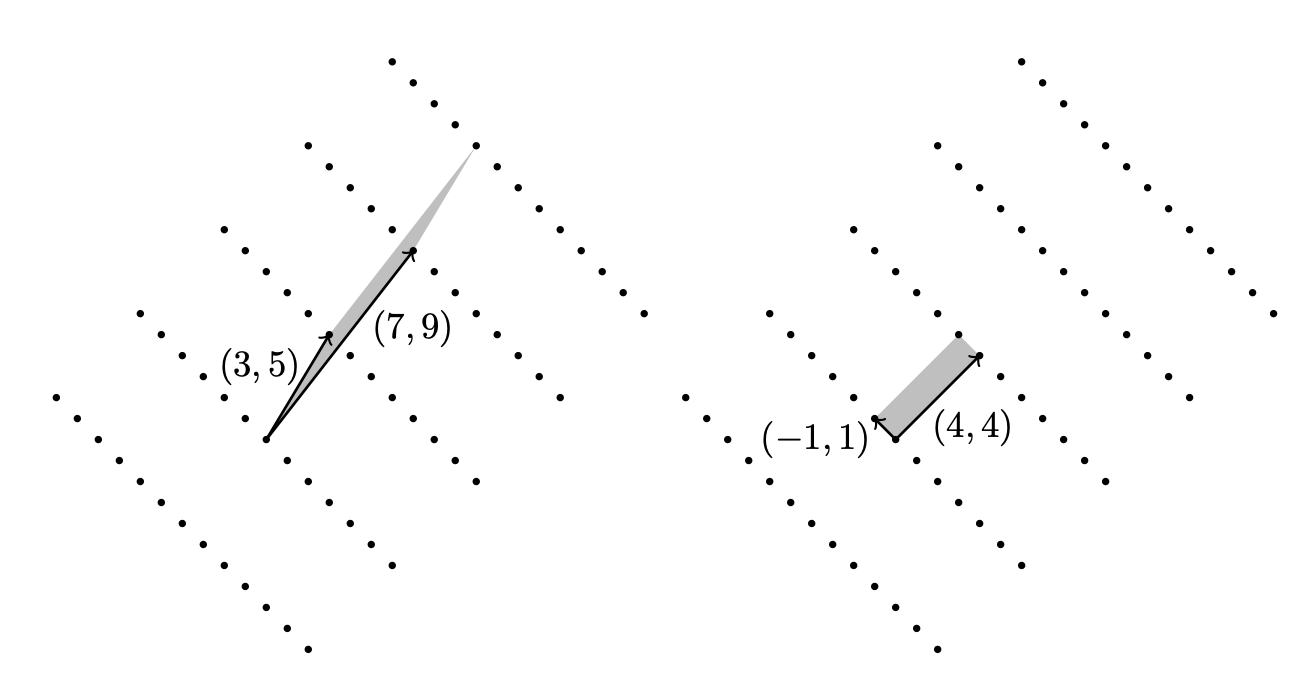# The Basic Concepts of LLL

I’ll not discuss LLL deeply. No proof. No code. Just concepts.

## Introduction

A lattice $$\Lambda$$ is a discrete subgroup generated by all the integer combinations of the vectors of some basis $$\bf{B}$$.

$\Lambda=\sum_{i=1}^{m}\mathbb{Z}b_i =\sum_{i=1}^{m}z_ib_i\text{ where }z_i\in \mathbb{Z},b_i\in\mathbb{R}^{n}$

Actually, a lattice can be represented with the matrix of its basis. It has a determinant, like matrix.

The determinant of a lattice $$\Lambda$$ ($$\det \Lambda$$) is an important numerical invariant of $$\Lambda$$.

If rank of lattice is not full, det 𝛬 is quite complicated but we will discuss only the full rank lattice.

$\det \Lambda=|\det [\bf{b_1b_2...b_n}]|$

Hadamard’s inequality : The determinant is less than or equal to the product of the norm of the basis vectors.

$\det \Lambda \leq \prod_{i=1}^{m}\|b_i\|$

Equality holds if and only if the vectors $$b_i$$ are pairwise orthogonal.

### Shortest vector problem

The shortest vector is the following : given a lattice $$\Lambda$$ find a shortest vector $$\bf{v}$$ among the set of vectors going from the zero element to any non-zero element $$x$$ in $$\Lambda$$.

The main theoretical result about this is the Minkowski’s theorem which gives us an upper bound for the shortest vector.

Minkowski’s theorem : Given a lattice $$\Lambda$$ of rank $$m$$, if $$\lambda$$ is the norm of the shortest vector then,

$\lambda \leq \frac{2}{\sqrt\pi}{\frac{m}{2}!}^{\frac{1}{m}}{\det\Lambda}^{\frac{1}{m}}$

where $$\frac{m}{2}!$$ is inductively defined by $$0!=1,\frac{1}{2}!=\frac{\sqrt{\pi}}{2}$$, and $$\frac{n}{2}!=\frac{n}{2}\cdot \frac{n-2}{2}!$$

## LLL Basis reduction

The idea of the basis reduction is to change a basis $$\bf{B}$$ of a lattice $$\Lambda$$ into a shorter basis $$\bf{B}'$$ such that $$\Lambda$$ remains the same.Basis reduction of rank 2 lattices is very easy. Just gram-schmidt and make orthogonal basis.

The LLL-reduction algorithm is a polynomial time lattice reduction algorithm. LLL is used to get an approximation of the shortest vector.

c-Reduced basis : A basis $$\{\bf{b_1, b_2, ...,b_m}\}$$ is said to be c-reduced if and only if its orthogonal basis $$\{\bf{b_1^\ast, b_2^\ast, ...,b_m^\ast}\}$$ verifies the following inequality for $$i=1$$ to $$m-1$$ :

${\|b_{i+1}^\ast\|}^{2}\geq \frac{\|b_{i}^\ast\|^{2}}{c}$

Theorem 1 : Given a lattice $$\Lambda$$ and its c-reduced basis $$\{\bf{b_1, b_2, ...,b_m}\}$$ with $$c\geq\frac{4}{3}$$, then it is near-orthogonal in the sense that:

$\prod_{i=1}^{m}{\|b_i\|}\leq c^{i-1}{\|b_i^\ast\|}^2$

Theorem 2 : If the basis $$\{\bf{b_1, b_2, ...,b_m}\}$$ is c-reduced and $$\lambda$$ is the shortest vector norm, then :

$\|b_1\|\leq c^{(m-1)/4}\det \Lambda^{\frac{1}{m}}\\ \|b_1\|\leq c^{(m-1)/2}\lambda$

Theorem 3 : For $$c\gt \frac{4}{3}$$, LLL finds a c-reduced basis in polynomial time.

Conclusion : So we can make reduced basis by LLL efficiently!

Factoring Polynomials with Rational Coefficients - A. K. Lenstra

LLL lattice basis reduction algorithm - Helfer Etienne